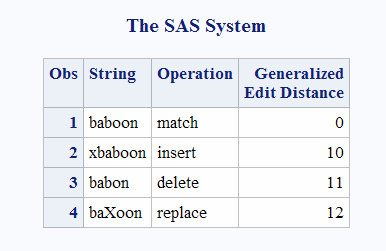# CALL COMPCOST Routine

Sets the costs of operations for later use by the COMPGED function

 Category: Character Restriction: Use with the COMPGED function Interaction: When invoked by the %SYSCALL macro statement, CALL COMPCOST removes quotation marks from its arguments. For more information, see .

## Syntax

CALL COMPCOST(operation-1, value-1 <,operation-2, value-2 …> );

### Required Arguments

#### operation

is a character constant, variable, or expression that specifies an operation that is performed by the COMPGED function.

#### value

is a numeric constant, variable, or expression that specifies the cost of the operation that is indicated by the preceding argument.

 Restriction Must be an integer that ranges from –32767 through 32767, or a missing value

## Details

### Computing the Cost of Operations

Each argument that specifies an operation must have a value that is a character string. The character string corresponds to one of the terms that is used to denote an operation that the COMPGED function performs. See Computing the Generalized Edit Distance to view a table of operations that the COMPGED function uses.
The character strings that specify operations can be in uppercase, lowercase, or mixed case. Blanks are ignored. Each character string must end with an equal sign (=). Valid values for operations, and the default cost of the operations are listed in the following table.
Operation
Default Cost
`APPEND=`
`very large`
`BLANK=`
`very large`
`DELETE=`
`100`
`DOUBLE=`
`very large`
`FDELETE=`
`equal to DELETE`
`FINSERT=`
`equal to INSERT`
`FREPLACE=`
`equal to REPLACE`
`INSERT=`
`100`
`MATCH=`
`0`
`PUNCTUATION=`
`very large`
`REPLACE=`
`100`
`SINGLE=`
`very large`
`SWAP=`
`very large`
`TRUNCATE=`
`very large`
If an operation does not appear in the call to the COMPCOST routine, or if the operation appears and is followed by a missing value, then that operation is assigned a default cost. A “very large” cost indicates a cost that is sufficiently large that the COMPGED function will not use the corresponding operation.
After your program calls the COMPCOST routine, the costs that are specified remain in effect until your program calls the COMPCOST routine again, or until the step that contains the call to COMPCOST terminates.

### Abbreviating Character Strings

You can abbreviate character strings. That is, you can use the first one or more letters of a specific operation rather than use the entire term. You must, however, use as many letters as necessary to uniquely identify the term. For example, you can specify the INSERT= operation as “in=”, and the REPLACE= operation as “r=”. To specify the DELETE= or the DOUBLE= operation, you must use the first two letters because both DELETE= and DOUBLE= begin with “d”. The character string must always end with an equal sign.

## Example

The following example calls the COMPCOST routine to compute the generalized edit distance for the operations that are specified.
```options pageno=1 nodate linesize=80 pagesize=60;
data test;
length String \$8 Operation \$40;
if _n_ = 1 then call compcost('insert=',10,'DEL=',11,'r=', 12);
input String Operation;
GED=compged(string, 'baboon');
datalines;
baboon  match
xbaboon insert
babon   delete
baXoon  replace
;
proc print data=test label;
label GED='Generalized Edit Distance';
var String Operation GED;
run;```
The following output shows the results.
Generalized Edit Distance Based on Operation